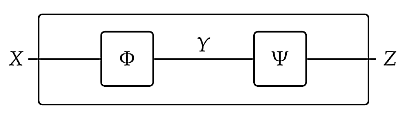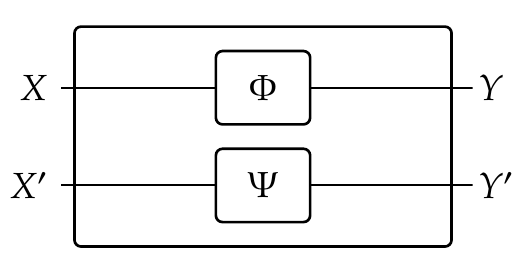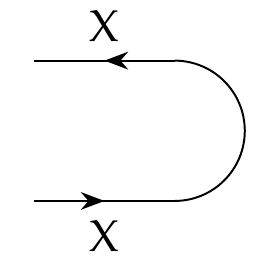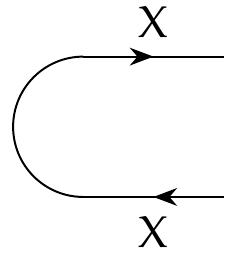I could move on to more realistic examples of collaborative design, but it would take a while to develop these, and I think our time will be better spent reaping the rewards of the mathematical work we've already done. So, for more on co-design, please read Chapter 4 of the book - and also this:

* Andrea Censi, [A mathematical theory of co-design](https://arxiv.org/abs/1512.08055).

Or ask me questions!

Right now I want to talk more about enriched profunctors. We've seen how to do lots of fun stuff with feasibility relations. We can 'compose' them, which describes running two processes in series:We can 'tensor' them, which describes running two processes in parallel:We can also create feedback loops using cups:and caps:These features are the key building blocks of co-design diagrams - but they also show up in diagrams of electrical circuits, and many other diagrams used by scientists and engineers! So, we should understand them more deeply.

For starters, feasibility relations are just a special case of \$$\mathcal{V}\$$-enriched profunctors, namely the case where \$$\mathcal{V} = \textbf{Bool}\$$. So, it's natural to ask if we can do all the same things with \$$\mathcal{V}\$$-enriched profunctors. And the answer is _yes!_

This is nice, because there's a variant of co-design diagrams where we use \$$\mathcal{V} = \textbf{Cost}\$$ instead of \$$\mathcal{V} = \textbf{Bool}\$$. In fact they were developed by the U.S. Navy starting in 1957 to help build nuclear submarines! They're called [PERT charts](https://en.wikipedia.org/wiki/Program_evaluation_and_review_technique), and they've been subsequently used for many other things. Of course, none of the practical people using PERT charts know they are dealing with enriched profunctors, and if you told them they probably wouldn't care. But still, this is a great example of how concepts that arise naturally in category theory can arise naturally in 'real life'.

So, let's take what we've done for feasibility relations, and do it for \$$\mathcal{V}\$$-enriched profunctors. It all works the same way.

We'll start by tensoring them.

In [Lecture 63](https://forum.azimuthproject.org/discussion/2295/lecture-63-chapter-4-composing-enriched-profunctors/p1) and [Lecture 64](https://forum.azimuthproject.org/discussion/2298/lecture-64-chapter-5-the-category-of-enriched-profunctors/p1) we saw that if \$$\mathcal{V}\$$ is any commutative quantale there's a category \$$\mathbf{Prof}\_\mathcal{V}\$$ with

* \$$\mathcal{V}\$$-enriched categories as objects,

* \$$\mathcal{V}\$$-enriched profunctors as morphisms,

and composition given by 'matrix multiplication':

$(\Psi\Phi)(x,z) = \bigvee_{y \in \mathrm{Ob}(\mathcal{Y})} \Phi(x,y) \otimes \Psi(y,z)$

whenever \$$\Phi \colon \mathcal{X} \nrightarrow \mathcal{Y} \$$ and \$$\Psi \colon \mathcal{Y} \to \mathcal{Z}\$$.

But \$$\mathbf{Prof}\_\mathcal{V}\$$ is better than a mere category: you can also _tensor_ objects in this category, and _tensor_ morphisms!

We saw how to tensor \$$\mathcal{V}\$$-enriched categories way back in [Lecture 62](https://forum.azimuthproject.org/discussion/2292/lecture-62-chapter-4-constructing-enriched-categories/p1). I wrote this tensor product with a times sign, but now it's better to use the symbol \$$\otimes\$$. Here's how it works: for any \$$\mathcal{V}\$$-enriched categories \$$\mathcal{X}\$$ and \$$\mathcal{Y}\$$, there is a \$$\mathcal{V}\$$-enriched category \$$\mathcal{X} \otimes \mathcal{Y}\$$ for which:

* an object is a pair \$$(x,y) \$$ where \$$x\$$ is an object of \$$\mathcal{X}\$$ and \$$y\$$ is an object of \$$\mathcal{Y}\$$, and

* we define

$(\mathcal{X} \times \mathcal{Y})((x,y), \, (x',y')) = \mathcal{X}(x,x') \otimes \mathcal{Y}(y,y') .$

Now let's figure out how to tensor \$$\mathcal{V}\$$-enriched profunctors! Suppose we have two of them, say

$\Phi \colon \mathcal{X} \nrightarrow \mathcal{Y}$

and

$\Psi \colon \mathcal{X'} \nrightarrow \mathcal{Y'}$

How can we define

$\Phi \otimes \Psi \colon X \otimes X' \nrightarrow Y \otimes Y' ?$

Well, we did this already for feasibility relations in [Lecture 66](https://forum.azimuthproject.org/discussion/2300/lecture-66-chapter-4-collaborative-design/p1), so we just copy that:

$(\Phi \otimes \Psi)((x,x'),(y,y')) = \Phi(x,y) \otimes \Psi(x',y') .$

You should imagine \$$\Phi \otimes \Psi\$$ as doing \$$\Phi\$$ and \$$\Psi\$$ in parallel:This ability to tensor things makes \$$\mathbf{Prof}_{\mathcal{V}}\$$ into a so-called ['monoidal category'](https://en.wikipedia.org/wiki/Monoidal_category). Instead of defining that - you can look up the definition if you care - I'll just get you to check some of the things a monoidal category should have.

First, we want tensoring to be associative. More precisely:

**Puzzle 224.** Suppose \$$\mathcal{X}, \mathcal{Y}\$$ and \$$\mathcal{Z}\$$ are \$$\mathcal{V}\$$-enriched categories. Show there is a \$$\mathcal{V}\$$-enriched profunctor

$\alpha\_{\mathcal{X}, \mathcal{Y},\mathcal{Z}} \colon (\mathcal{X} \otimes \mathcal{Y}) \otimes \mathcal{Z} \nrightarrow \mathcal{X} \otimes (\mathcal{Y} \otimes \mathcal{Z})$

which has an inverse.

There is just one answer to this puzzle, and this choice of \$$\alpha\_{\mathcal{X}, \mathcal{Y},\mathcal{Z}}\$$ is called the **associator**.

Remember, there's a \$$\mathcal{V}\$$-enriched category called \$$\mathbf{1}\$$. This has one object \$$0\$$ and \$$\mathbf{1}(0,0) = I \$$, where \$$I\$$ is the multiplicative identity in \$$\mathcal{V}\$$. Not surprisingly, this guy \$$\mathbf{1}\$$ is the unit for tensoring! More precisely:

**Puzzle 225.** Suppose \$$\mathcal{X}\$$ is a \$$\mathcal{V}\$$-enriched categories. Show there are \$$\mathcal{V}\$$-enriched profunctors

$\lambda_{\mathcal{X}} \colon \mathbf{1} \otimes \mathcal{X} \nrightarrow \mathcal{X}$

and

$\rho_{\mathcal{X}} \colon \mathcal{X} \otimes \mathbf{1} \nrightarrow \mathcal{X}$

both of which have inverses.

There's just one choice of each of these, unless you're a lot more sneaky than me. We call \$$\lambda_{\mathcal{X}}\$$ the **left unitor** and \$$\rho_{\mathcal{X}}\$$ the **right unitor**.

That's most of what we need for a monoidal category! Not everything: there are some subtleties. We want tensoring to define a functor \$$\otimes \colon \mathbf{Prof}\_{\mathcal{V}} \times \mathbf{Prof}\_{\mathcal{V}} \to \mathbf{Prof}\_{\mathcal{V}}\$$. And we need the associator and unitors to obey some equations.

But instead of scaring you with these details now, let me pound home the basic idea:

_Monoidal categories let us study processes that we can run in series and in parallel._

You already know dozens of monoidal categories, and now you know infinitely many more: all the categories \$$\mathbf{Prof}_{\mathcal{V}}\$$.

**Puzzle 226.** List a bunch of monoidal categories. Don't be afraid to guess: if a category has a good way of combining two objects to get a new object, and it seems kind of associative, chances are you've got a monoidal category.

**[To read other lectures go here.](http://www.azimuthproject.org/azimuth/show/Applied+Category+Theory#Chapter_4)**